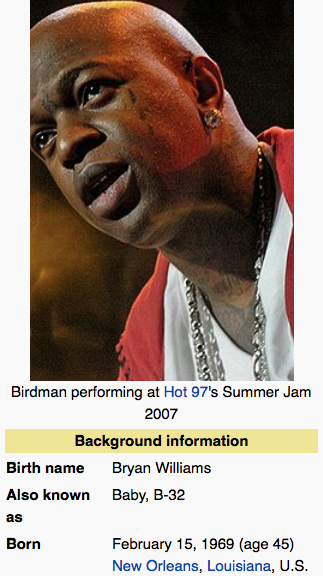## Wednesday, January 28, 2015

### 33 51 | Lil Wayne Sues Birdman for \$51-MillionDwayne = 27/72; Michael = 33/51; Carter 29/65; Dwayne Michael Carter = 89/188Lil = 15/33; Wayne = 23/68; Lil Wayne = 38/1019/27/1982 = 9+2+7+1+9+8+2 = 38; 9/27/82 = 9+27+82 = 118Bryan = 24/60; Williams = 35/44/98; Bryan Williams = 59/68/158Birdman = 34/70 (Bird = 33)2/15/1969 = 2+1+5+1+9+6+9 = 33; 2/15/69 = 2+15+69 = 86

Lil Wayne wants out from Cash Money records, and he is suing his 'boss' "Birdman" for \$51 million, the number of conspiracy.
• Cash Money = 3+1+1+8+4+6+5+5+7 = 40
• Cash Money = 3+1+10+8+4+6+5+5+7 = 49
• Silver = 1+9+3+22+5+9 = 49 (Lots of silver looking teeth around Cash Money records)
Also mentioned are "Drake" and "Nicki Minaj".
• Drake = 4+9+1+2+5 = 21
• Drake = 4+9+1+11+5 = 30
• Drake = 4+18+1+11+5 = 39
• Nicki Minaj = 5+9+3+2+9+4+9+5+1+1 = 48
• Nicki Minaj = 5+9+3+11+9+4+9+5+1+1 = 57
• Nicki Minaj = 14+9+3+11+9 = 46
• Minaj = 13+9+14+1+10 = 47
• Nicki Minaj = 93
Nicki Minaj and Drake both have life numbers of 31...
• 12/8/1982 = 1+2+8+1+9+8+2 = 31 (Nicki Minaj)
• 10/24/1986 = 1+0+2+4+1+9+8+6 = 31 (Drake)
In total, I think we're looking at a stage of actors, from Birdman to Lil Wayne, and this is just going through the motions as they're always being directed to go through.  At this point they're getting older, their stars are fading, and sadly for both men, they have some unfortunate life numbers and name Gematria.  With regards to Lil Wayne, Tupac and Notorious BIG both died on days with '38 numerology'.